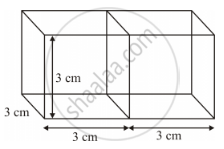Share

# Two Cubes Each of Volume 27 Cm3 Are Joined End to End to Form a Solid. Find the Surface Area of the Resulting Cuboid. - CBSE Class 10 - Mathematics

ConceptSurface Area of a Combination of Solids

#### Question

Two cubes each of volume 27 cm3 are joined end to end to form a solid. Find the surface area of the resulting cuboid.

#### Solution

Let the edge of each cube be a cm.

Volume of each cube = a3 cm3

It is given that the volume of each cube is 27 cm3

∴ a3 = 27= (3)3

⇒ a = 3

Thus, length of each edge of the cube = 3 cm

When two cubes are joined end-to-end, the solid obtained is a cuboid whose length, breadth and height are 6 cm, 3 cm and 3 cm respectively.

This can be diagrammatically shown as follows:Surface area of the cuboid = 2 (lb + bh + hl

= 2 (6 cm × 3 cm + 3 cm × 3 cm + 3 cm × 6 cm)

= 2 × 45 cm2

= 90 cm2

Thus, the surface area of the resulting cuboid is 90 cm2

Is there an error in this question or solution?

#### Video TutorialsVIEW ALL 

Solution Two Cubes Each of Volume 27 Cm3 Are Joined End to End to Form a Solid. Find the Surface Area of the Resulting Cuboid. Concept: Surface Area of a Combination of Solids.
S